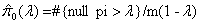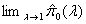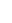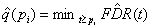Statistical test in ArrayXPath

A 2 by 2 table is constructed containing the two cluster memberships (within a certain cluster / out of the cluster) as row variables and pathway memberships (within the pathway / out of the pathway) as column variables. Since normal approximation is inappropriate in the pathway case (i.e. the contingency table often contains a cell with expected values less than 5), Fishers exact test is performed instead of the chi-square test. The p-value is defined as the sum of the probabilities of all tables whose probabilities are less than those of the observed table. To deal with the multiple testing problem, q-value is calculated following Storey's scheme. Whereas the p-value is a measure of significance in terms of the false positive rate, the q-value is a measure in terms of the false discovery rate(or FDR). FDR, here, is the expected proportion of false positive results among all rejected hypotheses multiplied by the probability of making at least one rejection. Firstly, the proportion of truly null genes is calculated from the given list of p-values. Secondly, the overall FDR is calculated as the expectation of the number of the false positive divided by the expectation of the number of the significant. Lastly, the q-value is extracted as the minimum of the FDR having the p-values less than threshold.

PNAS, vol. 100, no. 16, 9440-9445

1. ArrayXPath first searches pathway resources and maps an input list of genes (or gene products) to the corresponding nodes of pathways.
2. Secondly, to evaluate the statistical significance of the matches, ArrayXPath applies Fisher’s exact test for each match. The basic strategy is to enumerate all possible tables with the same margins as the observed table and to compute the exact probability for each table based on hyper-geometric distribution. Therefore, as recommended by the referee, we have appropriately applied a statistic based on hypergeometric distribution. The p-value is defined as the summation of the probabilities of all tables whose probabilities are smaller than the observed table. The null hypothesis is that the genes in the same cluster are randomly distributed across the biological pathways.
3. Thirdly, to deal with the problem of “multiple-hypothesis testing”, we calculated FDR (False Discovery Rate) as an error measurement. FDR is defined as the expected proportion of false positive results among all rejected hypotheses (multiplied by the probability of making at least one rejection). As the referee correctly pointed out, the accurate estimation of the proportion of the truly null hypotheses may not be an easy task. Basically, we followed the scheme of Storey et al.’ as follows.

Assuming that null p are uniformly distributed in the density plot of p-values, the proportion of truly null hypotheses (=π0) can be ‘conservatively’ estimated as the height of flat proportion of p exceeding a certain threshold value λ(16). Since most p values near 1 will be null,where m is the total number of hypotheses

will be an unbiased estimator of π0. To achieve the smallest bias,is used. Graphically, after the natural spline cube is fitted to the plot of π0 (λ) vs.  λ, the limiting plateau value is selected. Then FDR is calculated as the number of false positive hypotheses divided by the number of significant hypotheses using the π0 (λ). Finally, the q value for ith hypothesis is defined as the minimum FDR asWhen the p value is the minimum possible false positive rate, the q value is the minimum possible false discovery rate. Detailed algorithm can be found in Storey et al.